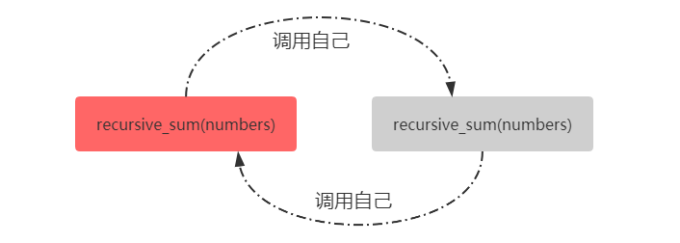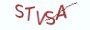Python基础教程

# 14.6.1 理解递归函数

Python也支持定义递归函数。所谓的递归函数，是指自己调用自己的函数。这里的调用不一定是直接调用，也可以是间接地调用。```# __desc__ = 定义一个简单的递归函数
def recursive_sum(numbers):
# 在函数内部直接调用自己
recursive_sum(numbers)``````# __desc__ = 定义一个简单的递归函数，并执行
def recursive_sum(numbers):
# 在函数内部直接调用自己
recursive_sum(numbers)

# 调用该递归函数
recursive_sum([1,2,3,4,5])```

Traceback (most recent call last):
File "demo.py", line 5, in <module>
recursive_sum([1,2,3,4,5])
File "demo.py", line 3, in recursive_sum
recursive_sum(numbers)
File "demo.py", line 3, in recursive_sum
recursive_sum(numbers)
File "demo.py", line 3, in recursive_sum
recursive_sum(numbers)
[Previous line repeated 996 more times]
RecursionError: maximum recursion depth exceeded

# 14.6.2 递归函数必须能够正常终止

```# __desc__ = 定义递归函数，逆序输出10到1之间的所有整数
def count_backwards(number):
# 当参数number大于0时，就输出number的值

if number > 0:
print(number)
number -= 1
# 不断调用自己，直到number小于等于0
count_backwards(number)

# 当参数number小于等于0时，函数将不再调用自己，此时会正常退出

# 执行这个递归函数
count_backwards(10)

""" 递归函数的输出为：
10
9
8
7
6
5
4
3
2
1
"""```

# 14.6.3 在递归函数中返回值

```# __desc__ = 定义一个非递归函数，将列表进行求和

def accumulate(numbers):
sum_of_numbers = 0
for _ in numbers:
sum_of_numbers += _
return sum_of_numbers

sum_of_numbers = accumulate([1,2,3,4])
# sum_of_numbers的输出为10```

```# __desc__ = 定义一个递归函数，将列表进行求和

def accumulate(numbers):
# 递归版本只需一行代码即可实现
return 0 if not numbers else numbers[-1]+accumulate(numbers[0:len(numbers)-1])

sum_of_numbers = accumulate([1,2,3,4])
# sum_of_numbers的输出为10```

(1) 该行代码最外层是一个三元运算结构：
return 0 if not numbers else numbers[-1]+accumulate(numbers[0:len(numbers)-1])
return 0 if not numbers

(2) 调用自己
else numbers[-1]+accumulate(numbers[0:len(numbers)-1])

numbers[-1]

accumulate(numbers[0:len(numbers)-1])

numbers[-1]+accumulate(numbers[0:len(numbers)-1])
(3) 递归执行

# 14.6.5 知识要点

(1) 所谓的递归函数，是指直接或间接地调用自身的函数。
(2) 在定义递归函数的时候必须设计函数的边界条件，一旦超出边界，就终止递归，否则递归函数会不断地执行，不断地消耗系统资源，直至被系统kill。

# 14.6.6 最具实力的小班培训

`(1) Python后端工程师高薪就业班，月薪11K-18K，免费领取课程大纲(2) Python爬虫工程师高薪就业班，年薪十五万，免费领取课程大纲(3) Java后端开发工程师高薪就业班，月薪11K-20K, 免费领取课程大纲(4) Python大数据分析，量化投资就业班，月薪12K-25K,免费领取课程大纲`## 注册账号